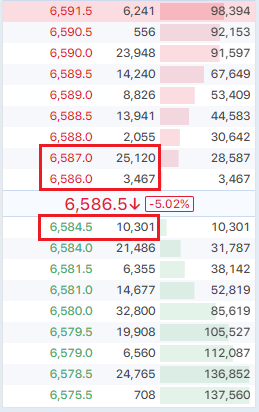BTSE 永续期货指数价格 = 大型交易所的平均现货流动中价

• 现货流动中价 = (买价 x 出售量 + 卖价 x 购买量) / (购买量 + 出售量)

• 为确保指数价格稳定，参考值将移除最高及最低值后再计算平均值
• 各币别所对应的交易所数量可能不同，详见附表说明

* 当参考价格有 5 个时，舍去最高及最低值后，取剩余 3 个计算平均值

BTSE 永续期货标记价格 = (现货流动中价 x 90% + BTSE 深度加权中价 x 10%)

• 现货流动中价 = (买价 x 出售量 + 卖价 x 购买量) / (购买量 + 出售量)
• BTSE 深度加权中价 = Average (深度加权买价, 深度加权卖价)
• 深度加权买价：委托列表中，买方出价最高的前 10,000 张订单的平均价格
• 深度加权卖价：委托列表中，卖方售价最低的前 10,000 张订单的平均价格

- 深度加权买价 = (6,584.5 x 10,000) / 10,000 = 6,584.5
- 深度加权卖价 = (6,586 x 3,467 + 6,587 x 6,533) / 10,000 = 6,586.65
- 深度加权中价 = (6,586.65 + 6,584.5) / 2 = 6,585.58• 标记价格与 BTSE 定期期货流动中价的价差不可超过 2%
• 当价差超过 2% 时，标记价格 = 指数价格

BTSE 定期期货指数价格 = 现货流动性中价 x (1 + 合理基差)

• 现货流动中价 = (买价 x 出售量 + 卖价 x 购买量) / (购买量 + 出售量)
• 合理基差 = 大型交易所的平均定期期货溢价 (或折价) 百分比

• 当 BTSE 定期期货到期日与参考的交易所相同时：
将以参考的交易所的定期期货溢价 (或折价) 百分比进行计算
• 当 BTSE 定期期货到期日与参考的交易所不同时：
取到期日最相近的二个参考交易所，辅以内插法 (Interpolation) 或外推法 (Extrapolation) 来计算溢价 (或折价) 百分比• 溢价 (或折价) 百分比生效条件：
• 参考来源的溢价 (或折价) 信息不可停摆
• 加权前后的指数价差不可超过 0.5%
• 未满足上述条件时，溢价 (或折价) 百分比 = 0

• 为确保指数价格稳定，参考值将移除最高及最低值后再计算平均值
• 各币别所对应的交易所数量可能不同，详见说明

* 当参考价格有 5 个时，舍去最高及最低值后，取剩余 3 个计算平均值

BTSE 定期期货标记价格 = BTSE 定期期货指数价格 x 90% + BTSE 定期期货流动中价 x 10%

• BTSE 定期期货指数价格：详见定期期货指数价格说明
• BTSE 定期期货流动中价 = (买价 x 出售量 + 卖价 x 购买量) / (购买量 + 出售量)

• 标记价格与 BTSE 定期期货流动中价的价差不可超过 2%
• 当价差超过 2% 时，标记价格 = 指数价格